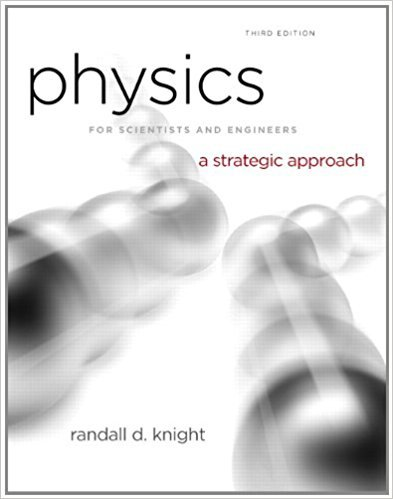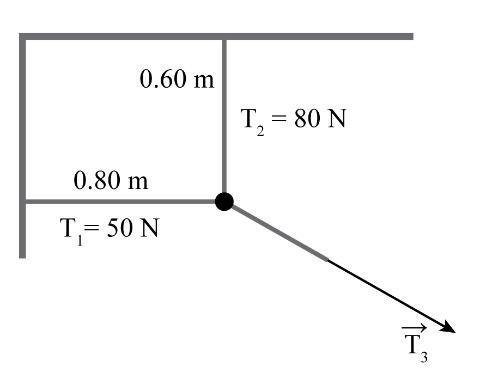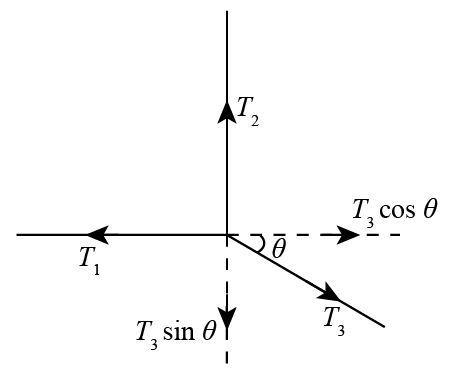×
Log in to StudySoup
Get Full Access to Physics For Scientists And Engineers: A Strategic Approach With Modern Physics - 3 Edition - Chapter 6 - Problem 2e
Join StudySoup for FREE
Get Full Access to Physics For Scientists And Engineers: A Strategic Approach With Modern Physics - 3 Edition - Chapter 6 - Problem 2e

Already have an account? Login here
×
Reset your password

# The three ropes in Figure P5.2 are tied to a small, veryISBN: 9780321740908 69

## Solution for problem 2E Chapter 6

Physics for Scientists and Engineers: A Strategic Approach with Modern Physics | 3rd Edition

• Textbook Solutions
• 2901 Step-by-step solutions solved by professors and subject experts
• Get 24/7 help from StudySoup virtual teaching assistantsPhysics for Scientists and Engineers: A Strategic Approach with Modern Physics | 3rd Edition

4 5 1 419 Reviews
13
5
Problem 2E

Problem 2E

The three ropes in Figure P5.2 are tied to a small, very light ring. Two of these ropes are anchored to walls at right angles with the tensions shown in the figure. What are the magnitude and direction of the tensionin the third rope?Step-by-Step Solution:

Problem 2E

The three ropes in Figure P5.2 are tied to a small, very light ring. Two of these ropes are anchored to walls at right angles with the tensions shown in the figure. What are the magnitude and direction of the tensionin the third rope?Step by Step Solution

Step 1 of 3

Given Data:

The tension in the horizontal rope is.

The tension in the vertical rope is.

The following is the free body diagram of the system.In order to determine the tension in the rope, we need to determine the net horizontal forces on the system.…… (1)

The expression of net vertical forces on the system is given as:……. (2)

Step 2 of 3

Step 3 of 3

##### ISBN: 9780321740908

The answer to “The three ropes in Figure P5.2 are tied to a small, very light ring. Two of these ropes are anchored to walls at right angles with the tensions shown in the figure. What are the magnitude and direction of the tension in the third rope?” is broken down into a number of easy to follow steps, and 45 words. Since the solution to 2E from 6 chapter was answered, more than 1798 students have viewed the full step-by-step answer. This full solution covers the following key subjects: ropes, figure, anchored, Angles, light. This expansive textbook survival guide covers 17 chapters, and 1439 solutions. This textbook survival guide was created for the textbook: Physics for Scientists and Engineers: A Strategic Approach with Modern Physics, edition: 3. Physics for Scientists and Engineers: A Strategic Approach with Modern Physics was written by and is associated to the ISBN: 9780321740908. The full step-by-step solution to problem: 2E from chapter: 6 was answered by , our top Physics solution expert on 08/30/17, 04:34AM.

Unlock Textbook Solution

Enter your email below to unlock your verified solution to:

The three ropes in Figure P5.2 are tied to a small, very